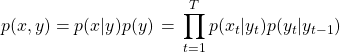# Machine Learning Interview Questions

``How do you handle missing data in an ML algorithm ?  What is the difference between word2Vec and Glove ?  How do you measure quality of Machine translation ?  What is the difference between deep learning and machine learning?  What are the commonly used activation functions ? When are they used.  What is the complexity of Viterbi algorithm ?  What are evaluation metrics for multi-class classification problem (like positive/negative/neutral sentiment analysis)  When are deep learning algorithms more appropriate compared to traditional machine learning algorithms?  You are given some documents and asked to find prevalent topics in the documents – how do you go about it ?  What is page rank algorithm ?  I have designed a 2 layered deep neural network for a classifier with 2 units in the hidden layer. I use linear activation functions with a sigmoid at the final layer. I use a data visualization tool and see that the decision boundary is in the shape of a sine curve. I have tried to train with 200 data points with known class labels and see that the training error is too high. What do I do ?  How do you deal with dataset imbalance in a problem like spam filtering ?  How to measure the performance of the language model ?  You have come up with a Spam classifier. How do you measure accuracy ?  What are some knowledge graphs you know. What is different between these ?  Suppose you are modeling text with a HMM, What is the complexity of finding most the probable sequence of tags or states from a sequence of text using brute force algorithm?  You want to find food related topics in twitter – how do you go about it ?  How do you train a hMM model in practice ?  Suppose you build word vectors (embeddings) with each word vector having dimensions as the vocabulary size(V) and feature values as pPMI between corresponding words: What are the problems with this approach and how can you resolve them ?  What is overfitting and underfitting ? Give examples. How do you overcome them?  Is the run-time of an ML algorithm important? How do I evaluate whether the run-time is OK?  What are knowledge graphs? When would you need a knowledge graph over say a database to store information?  What are the different ways of representing documents ?  What is the difference between supervised and unsupervised learning ?  What is speaker segmentation in speech recognition ? How do you use it ?  How can you increase the recall of a search query (on search engine or e-commerce site) result without changing the algorithm ?  What is PMI ?  I have used a 4 layered fully connected network to learn a complex classifier boundary. I have used tanh activations throughout except the last layer where I used sigmoid activation for binary classification. I train for 10K iterations with 100K examples  (my data points are 3 dimensional and I initialized my weights to 0 to begin with). I see that my network is unable to fit the training data and is leading to a high training error. What is the first thing I try ?  What are the advantages and disadvantages of using naive bayes for spam detection?  Can you give an example of a classifier with high bias and high variance?  How do you design a system that reads a natural language question and retrieves the closest FAQ answer?  How does KNN algorithm work ? What are the advantages and disadvantages of KNN ?  What is stratified sampling and why is it important ?  What are the different ways of preventing over-fitting in a deep neural network ? Explain the intuition behind each  What would you care more about – precision or recall for spam filtering problem?  If the average length of a sentence is 100 in all documents, should we build 100-gram language model ?  How will you build an auto suggestion feature for a messaging app or google search?  What are the different independence assumptions in hMM & Naive Bayes ?  Why do you typically see overflow and underflow when implementing an ML algorithms ?  With the maximum likelihood estimate are we guaranteed to find a global Optima ?  How do you generate text using a hMM model given below:What are popular ways of dimensionality reduction in NLP tasks ? Do you think this is even important ?  How many parameters are there for an hMM model?  What is negative sampling when training the skip-gram model ?  You are building a natural language search box for a website. How do you accommodate spelling errors?  What are some common tools available for NER ? Named Entity Recognition ?  ``